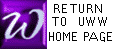PRACTICE USING FACTORING FORMULAS
NetScape 3.0+ or Internet Explorer 4.0+ is required

Suggestions for this self exam:
 [A] Study the 6 factoring formulas described in chapter 3. Each of these rules applies to a particular "FORM" of expression, so you must identify the "form" of your expression before choosing a formula to factor it.
 [B] Keep your mouse arrow to the side as you think. With NetScape 3.0 or better (as in most UW-W labs), move your mouse over the proper button (without clicking) to check your answer. For any browser, you may also click on the buttons, and factors are revealed at the correct button.

  To factor 10x2 - 30y3 , what formula is used FIRST ?    To see the factors, click on the correct button below.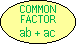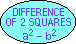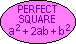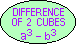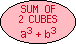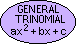To factor x2 + 10x + 25 , what formula is used FIRST ?    To see the factors, click on the correct button below.To factor 4x2 + 11x + 6 , what formula is used FIRST ?    To see the factors, click on the correct button below.To factor 8 - 27x6 , what formula is used FIRST ?    To see the factors, click on the correct button below.To factor 121x2 - y4 , what formula is used FIRST ?    To see the factors, click on the correct button below.To factor 4x2 + 16x + 16 , what formula is used FIRST ?    To see the factors, click on the correct button below.To factor 125x3 + 64 , what formula is used FIRST ?    To see the factors, click on the correct button below.To factor x(x - 2) - 5(x - 2) , what formula is used FIRST ?    To see the factors, click on the correct button below.To factor 6x4 + 23x2 + 20 , what formula is used FIRST ?    To see the factors, click on the correct button below.To factor 9x2 - 625 , what formula is used FIRST ?    To see the factors, click on the correct button below.To factor x4 - x , what formula is used FIRST ?    To see the factors, click on the correct button below.To factor x6+22x3+121 , what formula is used FIRST?    To see the factors, click on the correct button below.To factor (x + 5)2 - 4 , what formula is used FIRST ?    To see the factors, click on the correct button below.To factor x3+ax-3a-3x2 , what formula is used FIRST?    To see the factors, click on the correct button below.Return toMath 141Home Page